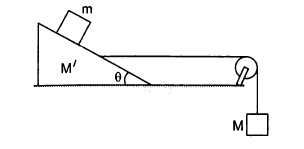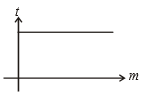For a rocket moving in free space, the fraction of mass to be disposed of Off to attain a speed equal to two times the exhaust speed is given by (given ${\mathrm{e}}^{2}$ = 7.4)

1. 0.40

2.  0.37

3.  0.50

4.  0.86

Subtopic:  Variable Mass System |
To view explanation, please enrol in the course below.
NEET 2021 - Achiever Batch - Aryan Raj Singh

Difficulty Level:

The length of a spring is  when stretched with a force of 4 N and 5 N respectively. Its natural length is?

(1) ${l}_{2}+{l}_{1}$

(2) $2\left({l}_{2}-{l}_{1}\right)$

(3) $5{l}_{1}-4{l}_{2}$

(4) $5{I}_{2}-4{l}_{1}$

Subtopic:  Spring Force |
To view explanation, please enrol in the course below.
NEET 2021 - Achiever Batch - Aryan Raj Singh

Difficulty Level:

A simple pendulum hangs from the roof of a train moving on horizontal rails. If the string is inclined towards the front of the train, then train is-

1. Moving with constant velocity

2. In accelerated motion

3. In retarded motion

4. At rest

Subtopic:  Application of Laws |
To view explanation, please enrol in the course below.
NEET 2021 - Achiever Batch - Aryan Raj Singh

Difficulty Level:

The value of M of the hanging block is in the figure, which will prevent the smaller block (m=1 kg) from slipping over the triangular block. All the surfaces are smooth and string and pulley are ideal. (Given: M'=4 kg and $\theta ={37}^{0}$)1. 12 kg

2. 15 kg

3. 10 kg

4. 4 kg

Subtopic:  Pseudo Force |
To view explanation, please enrol in the course below.
NEET 2021 - Achiever Batch - Aryan Raj Singh

Difficulty Level:

A lighter body and heavier body both are moving with same momentum and applied same retarding force. Their stoping distances are  respectively. Then the correct relation between -

1. ${s}_{1}>{s}_{2}$

2. ${s}_{1}<{s}_{2}$

3. ${s}_{1}={s}_{2}$

4. None of these

Subtopic:  Newton's Laws |
To view explanation, please enrol in the course below.
NEET 2021 - Achiever Batch - Aryan Raj Singh

Difficulty Level:

A plastic box of mass 5 kg is found to accelerate up at the rate of g / 6, when placed deep inside the water. How much sand should be put inside the box so that it may accelerate down at the rate of g/6?

1.  2kg

2.  3kg

3.  4kg

4.  5kg

Subtopic:  Application of Laws |
To view explanation, please enrol in the course below.
NEET 2021 - Achiever Batch - Aryan Raj Singh

Difficulty Level:

Let $\theta$ denote the angular displacement of a simple pendulum oscillating in a vertical plane. If the mass of bob is m, the tension in the string is mg cos $\theta$-

1.  always

2.  never

3.  at extreme

4.  at mean position

Subtopic:  Non Uniform Vertical Circular Motion |
To view explanation, please enrol in the course below.
NEET 2021 - Achiever Batch - Aryan Raj Singh

Difficulty Level:

In a uniform circular motion, which of the following quantity is not constant

1. Angular momentum

2. Speed

3. Kinetic energy

4. Momentum

Subtopic:  Uniform Circular Motion |
To view explanation, please enrol in the course below.
NEET 2021 - Achiever Batch - Aryan Raj Singh

Difficulty Level:

The graph for stopping time (t) versus mass (m) is shown in the figure. Then which one of the pair is given1.  Momentum, force

2.  K.E., force

3.  Velocity, force

4.  None of these

Subtopic:  Application of Laws |
To view explanation, please enrol in the course below.
NEET 2021 - Achiever Batch - Aryan Raj Singh

Difficulty Level:

A metal ring of mass m and radius R is placed on smooth horizontal table and is set rotating about its own axis in such a way that each part of the ring moves with a speed v. Tension in the ring is:

1. $\frac{m{v}^{2}}{R}$

2. $\frac{m{v}^{2}}{2R}$

3. $\frac{m{v}^{2}}{2\mathrm{\pi }R}$

4. None of these

Subtopic:  Uniform Circular Motion |
To view explanation, please enrol in the course below.
NEET 2021 - Achiever Batch - Aryan Raj Singh

Difficulty Level: